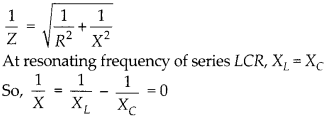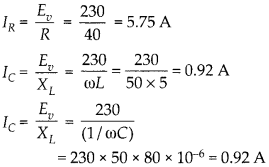Enlightened

# Question 17: NCERT Solutions for 12th Class Physics: Chapter 7-Alternating Current

• 0

Question 17: NCERT Solutions for 12th Class Physics: Chapter 7-Alternating Current

Keeping the source frequency equal to the resonating frequency of the series LCR circuit, if the three elements, L, C and R are arranged in parallel, show that the total current in the parallel LCR circuit is minimum at this frequency. Obtain the current rms value in each branch of the circuit for the elements of frequency. Source has emf 230 V and L = 5.0 H, C = 80 μF, ff = 40 Ω2.

Share

1. Solution:

impedance of  R and X in parallel is given byThus, impedance Z = R and will be maximum. Hence, in parallel resonant circuit, current is minimum at resonant frequency. Current through circuit elementsSince, IL and IC are opposite in phase, so net current,Check the complete chapter with solutions.

NCERT Solutions for 12th Class Physics: Chapter 7-Alternating Current

• 0